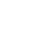Javascript is required
1.
A. Majeed Jasim‎, “New analytical study for nanofluid between two non-parallel plane walls (Jeffery-Hamel Flow),” J. Appl. Comput. Mech., vol. 7, no. 1, pp. 213–224, 2021. [Google Scholar] [Crossref]
2.
T. H. Kuehn and R. J. Goldstein, “An experimental and theoretical study of natural convection in the annulus between horizontal concentric cylinders,” J. Fluid Mech., vol. 74, no. 4, pp. 695–719, 1976. [Google Scholar] [Crossref]
3.
M. A. Medebber, N. Retiel, A. Aissa, and M. El Ganaoui, “Transient numerical analysis of free convection in cylindrical enclosure,” in International Conference on Materials & Energy, (ICOME’17 and ICOME’18). Tiajin, China: EDP Sciences, 2018. [Google Scholar]
4.
Y. T. Tsui and B. Tremblay, “On transient natural convection heat transfer in the annulus between concentric, horizontal cylinders with isothermal surfaces,” Int. J. Heat Mass Transfer, vol. 27, no. 1, pp. 103–111, 1984. [Google Scholar] [Crossref]
5.
L. Zhang, Y. Hu, and M. Li, “Numerical study of natural convection heat transfer in a porous annulus filled with a Cu-nanofluid,” Nanomaterials, vol. 11, no. 4, p. 990, 2021. [Google Scholar] [Crossref]
6.
L. Crawford and R. Lemlich, “Natural convection in horizontal concentric cylindrical annuli,” Ind. Eng. Chem. Fundam., vol. 1, no. 4, pp. 260–264, 1962. [Google Scholar] [Crossref]
7.
L. R. Mack and E. H. Bishop, “Natural convection between horizontal concentric cylinders for low Rayleigh numbers,” Q. J. Mech. Appl. Math., vol. 21, no. 2, pp. 223–241, 1968. [Google Scholar] [Crossref]
8.
I. Pop, D. B. Ingham, and P. Cheng, “Transient natural convection in a horizontal concentric annulus filled with a porous medium,” J. Heat Transfer, vol. 114, no. 4, pp. 990–997, 1992. [Google Scholar] [Crossref]
9.
A. K. Hassan and J. M. Al-lateef, “Numerical simulation of two dimensional transient natural convection heat transfer from isothermal horizontal cylindrical annuli,” J. Eng., vol. 13, no. 2, pp. 1429–1444, 2007. [Google Scholar]
10.
S. Touzani, A. Cheddadi, and M. T. Ouazzani, “Natural Convection in a Horizontal Cylindrical Annulus with Two Isothermal Blocks in Median Position: Numerical Study of Heat Transfer Enhancement,” J. Appl. Fluid Mech., vol. 13, no. 1, pp. 327–334, 2020. [Google Scholar] [Crossref]
11.
A. S. J. Al-Saif and J. Takia Ahmed  Al-Griffi, “Analytical simulation for transient natural convection in a horizontal cylindrical concentric annulus,” Journal of Applied and Computational Mechanics, vol. 7, no. 2, pp. 621–637, 2021. [Google Scholar] [Crossref]
12.
M. H. Matin, O. Mahian, and S. Wongwises, “Nanofluids flow between two rotating cylinders: Effects of thermophoresis and brownian motion,” J. Thermophys. Heat Transfer, vol. 27, no. 4, pp. 748–755, 2013. [Google Scholar] [Crossref]
13.
A. S. Rehan and A. Izhar, “Theoretical analysis of unsteady flow between two stretching cylinders with variable physical properties,” Global Sci. J., vol. 8, no. 11, pp. 772–784, 2020. [Google Scholar]
14.
C. Shu, H. Xue, and Y. D. Zhu, “Numerical study of natural convection in an eccentric annulus between a square outer cylinder and a circular inner cylinder using DQ method,” Int. J. Heat Mass Transfer, vol. 44, no. 17, pp. 3321–3333, 2001. [Google Scholar] [Crossref]
15.
C. Shu and Y. D. Zhu, “Efficient computation of natural convection in a concentric annulus between an outer square cylinder and an inner circular cylinder,” Int. J. Numer. Meth. Fluids, vol. 38, no. 5, pp. 429–445, 2002. [Google Scholar] [Crossref]
16.
J. H. He, “A coupling method of a homotopy technique and a perturbation technique for non-linear problems,” Int. J. Non-Linear Mech., vol. 35, no. 1, pp. 37–43, 2000. [Google Scholar] [Crossref]
17.
J. H. He, “The homotopy perturbation method for nonlinear oscillators with discontinuities,” Appl. Math. Comput., vol. 151, no. 1, pp. 287–292, 2004. [Google Scholar] [Crossref]
18.
J. H. He, “Homotopy perturbation technique,” Comput. Methods Appl. Mech. Eng., vol. 178, no. 3–4, pp. 257–262, 1999. [Google Scholar] [Crossref]
19.
J. Singh and Y. S. Shishodia, “A reliable approach for two-dimensional viscous flow between slowly expanding or contracting walls with weak permeability using sumudu transform,” Ain Shams Eng. J., vol. 5, no. 1, pp. 237–242, 2014. [Google Scholar] [Crossref]Search
OutlineOpen Access
Research article
FLT-HPM for Two-dimensional Transient Natural Convection in a Horizontal Cylindrical Concentric Annulus
Department of Mathematics, College of Education for Pure Science, Basrah University, 61001 Basrah, Iraq
Power Engineering and Engineering Thermophysics
|
Volume 2, Issue 3, 2023
|
Pages 120-138
Revised: 04-24-2023,
Accepted: 05-17-2023,
Available online: 08-16-2023View Full Article|Download PDF
Abstract:

A hybrid procedure FLT-HPM was proposed in this study, by combining the homotopy perturbation method (HPM) with Fourier transform and Laplace transform which aimed to find an approximate analytical solution to the problem of two-dimensional transient natural convection in a horizontal cylindrical concentric annulus bounded by two isothermal surfaces. The effect of the Grashof number, Prandtl number, and the radius ratio on fluid flow (air) and heat transfer with different values awreas discussed. Moreover, the velocity distributions and the mean Nusselt numbers were studied, and the Nusselt numbers were used to represent local and general heat transfer rates. Finally, the convergence of FLT-HPM was tested theoretically through the proof of some theorems. In addition, these theorems were applied to the results of the new solutions obtained using FLT-HPM.

Keywords: FLT-HPM, Homotopy perturbation method, Fourier transform, Laplace transform, Natural convection, Cylindrical annulus

Cite this:
APA Style
IEEE Style
BibTex Style
MLA Style
Chicago Style
Abdulameer, Y. A. & Al-Saif, A. J. A. (2023). FLT-HPM for Two-dimensional Transient Natural Convection in a Horizontal Cylindrical Concentric Annulus. Power Eng. Eng Thermophys., 2(3), 120-138. https://doi.org/10.56578/peet020301
ccTable 1. Absolute error comparison of $\psi(r, \theta)$ between FLT-HPM, YT-HPM and HPM with $G r=1000, P r=0.7$ and $R=2$Views: 130